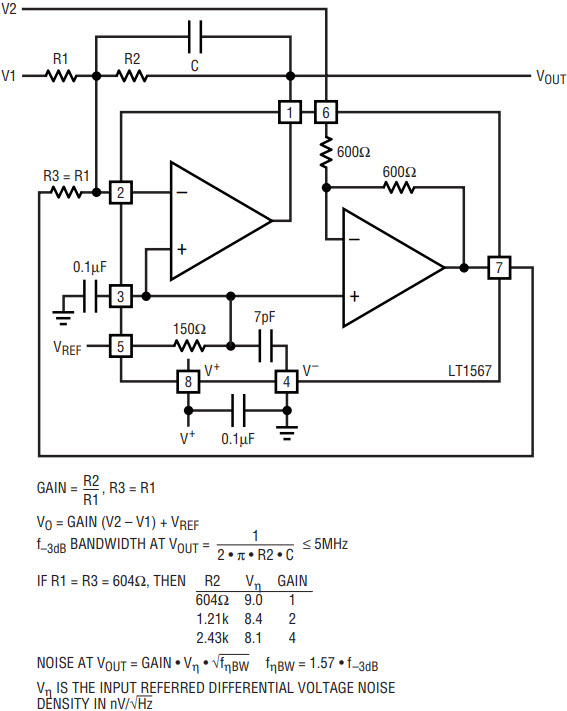# Design Low Noise Differential Circuits Using the LT1567 Dual Amplifier Building Block

### Introduction

Many communications systems use differential, low level (400mV – 1V peak-to-peak), analog baseband signals, where the baseband circuitry operates from with a single low voltage power supply (5V to 3V). Any differential amplifier circuit used for baseband signal conditioning must have very low noise, and an output voltage swing that includes most of the power supply range for maximum signal dynamic range. The LT1567, a low noise operational amplifier (1.4nV/√Hz voltage noise density) and a unity-gain inverter, is an excellent analog building block (see Figure 1) for designing low noise differential circuits. The typical gain bandwidth of the LT1567 amplifier is 180MHz and op amp slew rate is sufficient for signal frequencies up to 5MHz. The LT1567 operates from 2.7V to 12V total power supply. The output voltage swing is guaranteed to be 4.4V and 2.6V peak-to-peak, at 1k load with a single 5V and 3V power supply respectively. The LT1567 is available in a tiny MS8 surface mount package.Figure 1. LT1567 analog building block.

### A Single-Ended To Differential Amplifier

Figure 2 shows a circuit for generating a differential signal from a single-ended input. The differential output noise is a function of the noise of the amplifiers, the noise of resistors R1 and R2 and the noise bandwidth. For example, if R1 and R2 are each 200Ω, the differential voltage noise density is 9.5nV/√Hz and in a 4MHz noise bandwidth the total differential noise is 19µVRMS (with a low level 0.2VRMS differential signal, the signal-to-noise ratio is an excellent 80.4dB). The voltage on Pin 5 (VREF) provides flexible DC bias for the circuit and can be set by a voltage divider or a reference voltage source (with a single 3V power supply, the VREF range is 0.9V ≤ VREF ≤ 1.9V). In a single supply circuit, if the input signal is DC coupled, then an input DC voltage (VINDC) is required to bias the input within the circuit’s linear region. If VINDC is within the VREF range, then VREF can be equal to VINDC and the output DC common mode voltage (VOUTCM) at VO1 and VO2 is equal to VREF. To maximize the unclipped LT1567 output swing however, the DC common mode output voltage must be set at V+/2. In addition, the input signal can be AC coupled to the circuit’s input resistor R1 and VREF set to the DC common mode voltage required by any following circuitry (for example the input of an I and Q modulator).Figure 2. A single-ended input to differential output amplifier.

### A Differential Buffer/Driver

Figure 3 shows an LT1567 connected as a differential buffer. The differential output voltage noise density is 7.7nV/√Hz. The differential buffer circuit of Figure 3, translates the input common mode DC voltage (VINCM) to an output common mode DC voltage (VOUTCM) set by the VREF voltage (VOUTCM = 2 • VREF – VINCM). For example, in a single 5V power supply circuit, if VINCM is 0.5V and VREF is 1.5V then VOUTCM is 2.5V.Figure 3. A differential input and output buffer/driver.

### A Differential to Single-Ended Amplifier

Figure 4 shows a circuit for converting a differential input to a single-ended output. For a gain equal to one (R1 = R2 = 604Ω and VOUT = V2 – V1) the input referred differential voltage noise density is 9nV/√Hz and differential input signal-to-noise ratio is 80.9dB with 0.1VRMS input signal in a 4MHz noise bandwidth. The input AC common mode rejection depends on the matching of resistors R1 and R3 and the LT1567 inverter gain tolerance (common mode rejection is at least 40dB up to 1MHz with one percent resistors and two percent inverter typical gain tolerance). If the differential input is DC coupled, then VREF must be set equal to input common mode voltage (VINCM) (if VREF is greater than VinCM then a peak voltage on Pin 7 may exceed the output voltage swing limit). The DC voltage at the amplifier’s output (VOUT, Pin 1) is VREF.Figure 4. A differential input-to-single-ended output amplifier.

### Conclusion

With one LT1567 and two or three resistors, it is easy to design low noise, differential circuits for signals up to 5MHz. The LT1567 can also be used to make of low noise second and third order lowpass filters and second order bandpass filters with differential outputs. See www.analog.com for a spreadsheet-based design tool for just this purpose.